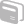Activities

•• Subject Area

• Maths: Number: Number
• Maths: Algebra: Algebra

• Author14-16

2 Hours

• TI-Nspire™

TI-Nspire™

Sums of Series

Activity Overview

This activity is a challenging investigation of the sum of a series of fractions. As well as using the calculator application to explore the sum, learners are introduced to the sigma notation and encouraged to graph the sequence of nth sums.

During the Activity

Objective: to explore series and become familiar with the sigma notation.

After the Activity

Further exploration of series e.g. arithmetic and geometric and associated notation.